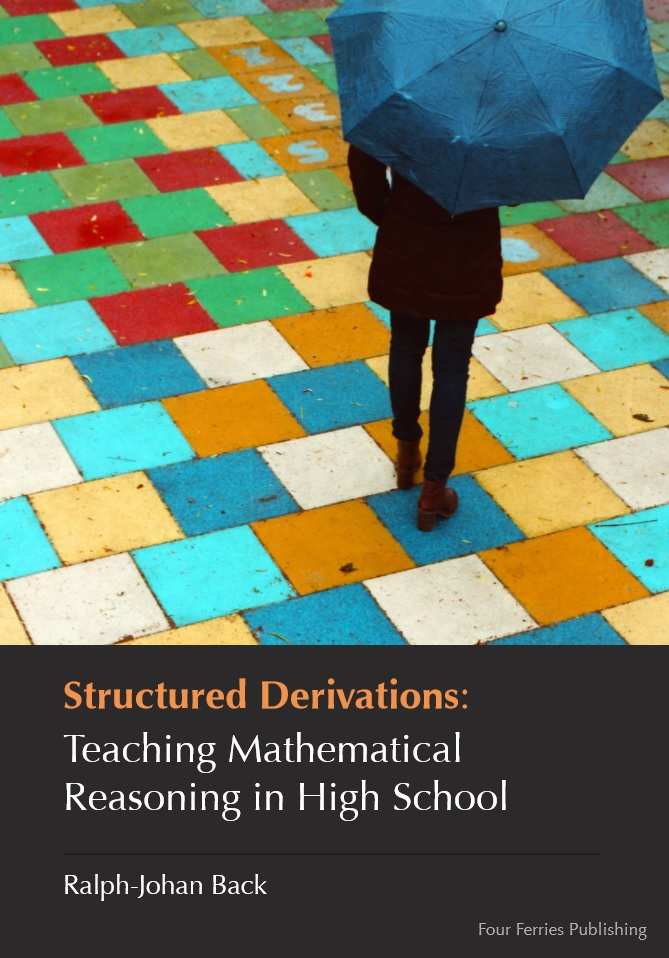# Structured Derivations: Teaching Mathematical Reasoning in High School (2015)Structured Derivations: Teaching Mathematical Reasoning in High School (2015)
1587 Downloads
Mathematics is based on proofs. The proof shows the logical reasoning behind a theorem, allows us to understand the meaning of it, its limitations and its consequences. Without a proof, a theorem is like magic; with a proof it is (sometimes more, sometimes less) self evident. But proofs are considered difficult in mathematics education of today, in particular at secondary level, and are therefore often avoided in teaching. When proofs are given, they are often informal and the underlying logic is not explicated. The purpose of this book is to put forward an alternative method for teaching mathematics at secondary and tertiary level that reintroduces proofs and careful argumentation as the solid basis for mathematics education. The method, structured derivations, is essentially a format for presenting mathematical arguments (calculations, derivations, proofs, problem solutions, etc). The format is designed to show the overall structure of the argument, while at the same time allowing a detailed inspection of each step in the argument. The method does not put any restrictions on the mathematical domain where the argumentation is carried out, nor on the level of detail or mathematical rigor of the argumentation. Hence, structured derivations can be used in any area of mathematics, and at any level of education. The focus in this book is on how to use structured derivations in teaching mathematics at high school level.
Size: 6 MB
Posted in .